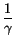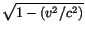Next: Lorentz Transformations and the Up: Special Relativity Previous: Time Dilation

# Length Contraction

You may have spotted a potential problem with the above discussion. From the muon's point of view, the decay process is not slowed down. The muon thinks it only lives the usual 2 microseconds so it should be impossible for it to travel over 4 kilometers before decaying. It would have to travel at almost 7 times the speed of light! The resolution should not be too surprising at this stage: if time is relative, then distance must also be relative. Consistency demands that the muon sees itself as traveling a distance of only v x t', where t' is the time elapsed in its frame, and v = .99c is its velocity. Thus, it thinks that it travels only the expected 600 meters before decaying. Since the muon is created near the top of the Earth's atmosphere, and decays somewhere near the bottom, it must conclude that the atmosphere of the Earth is only about 600 meters deep, or about one seventh of what someone on Earth measures it to be.

This then is a general feature of special relative. The length of objects appear contracted when viewed from a moving frame. The apparent length (L') is proportional to the actual length, L, as measured in the objects frame of reference. The constant of proportionality is the inverse of the time dilation factor. In terms of equations:

 L' =L =L (11.7)

Clearly the apparent length is always less than the actual length, so this effect is called length contraction. As with time dilation, the situation must be symmetrical. If objects, such as the atmosphere, that are at rest relative to the Earth appear contracted to the muon, then objects moving with the muon must appear shortened by the same factor when viewed from the Earth. Consider for example the size of the muon itself. Muons are very tiny particles and have virtually no extent, but let us for the sake of illustration assume that as measured in the muon's frame of reference, it has a width of one centimeter. Length contraction tells us that the size of a muon moving at 0.99c will appear contracted to one seventh of its true size (or just over a millimeter) when viewed by an Earthbound observer. Thus, as expected, lengths of objects attached to the muon appear contracted as seen from Earth, whereas lengths of objects moving with the Earth appear contracted as measured in the muon's frame.

One last thing to emphasize is that the entire discussion so far refers only to one dimensional motion. Length contraction applies only to lengths along the direction of motion. It is of course possible to apply the postulates of Special Relativity to three dimensional motion, but the analysis gets considerably more complicated, and no essential new features emerge.Next: Lorentz Transformations and the Up: Special Relativity Previous: Time Dilation
modtech@theory.uwinnipeg.ca
1999-09-29